# Mathematical explanation for Linear Regression working

Suppose we are given a dataset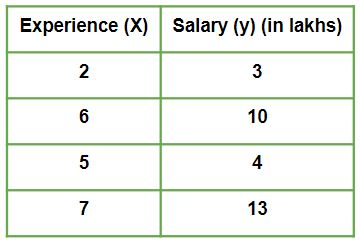Given is a Work vs Experience dataset of a company and the task is to predict the salary of a employee based on his / her work experience.
This article aims to explain how in reality Linear regression mathematically works when we use a pre-defined function to perform prediction task.
Let us explore how the stuff works when Linear Regression algorithm gets trained.
.
Iteration 1 – In the start, θ0 and θ1 values are randomly choosen. Let us suppose, θ0 = 0 and θ1 = 0.

• Predicted values after iteration 1 with Linear regression hypothesis.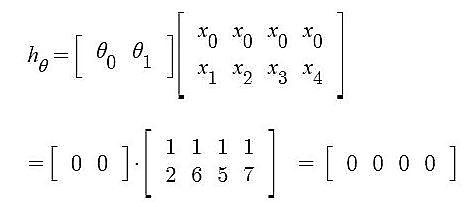• Cost Function – Error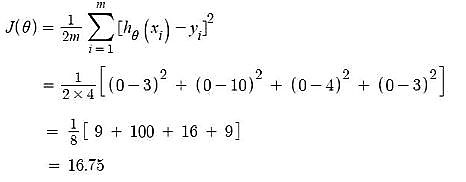• Gradient Descent – Updating θ0 value
Here, j = 0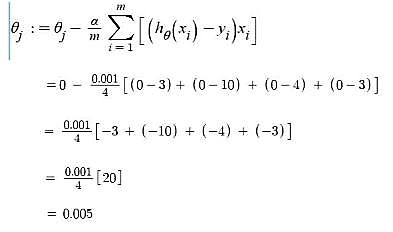• Gradient Descent – Updating θ1 value
Here, j = 1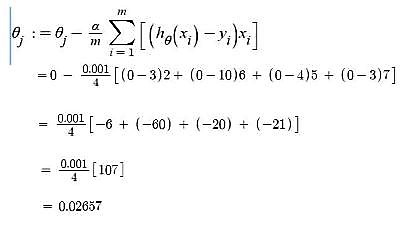Iteration 2 – θ0 = 0.005 and θ1 = 0.02657

• Predicted values after iteration 1 with Linear regression hypothesis.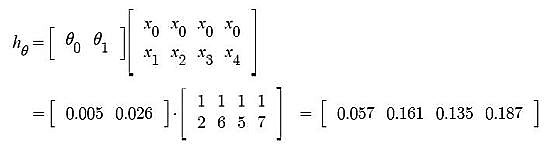• Now, similar to iteration no. 1 performed above we will again calculate Cost function and update θj values using Gradient Descent.
We will keep on iterating until Cost function doesn’t reduce further. At that point, model achieves best θ values. Using these θ values in the model hypothesis will give the best prediction results.

My Personal Notes arrow_drop_upIf you like GeeksforGeeks and would like to contribute, you can also write an article using contribute.geeksforgeeks.org or mail your article to contribute@geeksforgeeks.org. See your article appearing on the GeeksforGeeks main page and help other Geeks.

Please Improve this article if you find anything incorrect by clicking on the "Improve Article" button below.